# The moons dataset and decision surface graphics in a Jupyter environment – VI – Kernel-based SVC algorithms

We continue with our article series on the moons dataset as an entry point into „Machine Learning“:

The moons dataset and the related classification problem posed interesting challenges for us as beginners in ML:

Most people starting with ML have probably studied the topic of linear classification to separate distinct data sets by a linear decision surface (hyperplane) on data (y, X) with X=(x1,x2) defining points in a 2-dim space.

The SVM-approach studied in this article series follows a so called „soft-margin“ classification: It tries to maximize the distances of the decision surface to the data points whilst it at the same time tries to reduce the number of points which violate the separation, i.e. points which end up on the wrong side of the decision hyperplane. This approach is controlled by so called hyper-parameters as a parameter „C“ in the LinearSVC algorithm. If we just used LinearSVC on the original (x1,x2) data plane the hyperplane would be some linear line.

Unfortunately, for the moons dataset we must to overcome the fundamental problem that a linear classification approach to define a decision surface between the two data clusters is insufficient. (We have confirmed this in our last article by showing that even a quadratic approach does not give any reasonable decision surface.) We circumvented this problem by a trick – namely a polynomial extension of the parameter space (x1,x2) via a SciKit-Learn function „PolynomialFeatures()“.

We also had to tackle the technical problem of writing a simple Python class for creating plots of a decision surface in a 2 dim parameter space (x1,x2). After having solved this problem in the last articles it became easy to apply different or differently parameterized algorithms to the data set and display the results graphically. The functionalities of the SciKit and numpy libraries liberated us from dealing with complicated mathematical tasks. We also saw that using the „Pipeline“ function helped to organize the sequential operations on the data sets – here transforming data, scaling data and training of a chosen algorithm on the data – in a very comfortable way.

In this article we want to visualize results of some other SVM approaches, which make use of the called „Kernel trick“. We shall explain this briefly and then use functions provided by SciKit to perform further experiments. On the Python side we shall learn, how to measure required computational times of the different algorithms.

# Artificial polynomial feature extension

So far we moved
around the hurdle of non-linearity in combination with LinearSVC by a costly trick: We have extended the original 2-dimensional parameter space X(x1, x2) of our data points [y, X] artificially by more X-dimensions. We proclaimed that the distribution of data points is actually given in a multidimensional space constructed by a polynomial transformation: Each new and additional dimension is given by a term f*(x1**n)*(x2**m) with n+m = D, the degree of a polynomial function of x1 and x2.

This works as if the results „y“ depended on further properties expressed by polynomial combinations of x1 and x2. Note that a 2-dim space (x1,x2) thus may be transformed into a 5-dimensional space with axes for x1, x2, x1**2, a*x1*x2, x**2. A data point (x1,x2) is transformed to a vector P(x1,x2) = [p_1=x1, p_2=x2, p_3=x1**2, p_4=a*x1*x2, p_5=x2**2]. Actually, for a broad class of problems it is enough to look at the 3-dim transformation space P([x1,x2])=[p_1=x1**2, p_2=f*x1*x2, p_3=x2**2].

In such a higher dimensional space we might actually find a „linear“ hyperplane which allows for a suitable separation for the data clusters belonging to 2 different values of y=y(x1,x2) – here y=0 and y=1. The optimization algorithm then determines a suitable parameter vector (Theta= [theta_0, theta_1, … theta=n]), describing an optimal linear surface with respect to the distance of the data points to this surface. If we omit details then the separation surface is basically described by some scalar product of the form

theta_0*1 + theta1*p_1 + theta2*p_2 + … + theta_D * p_D = const.

Our algorithm calculates and optimizes the required theta-values.

Note that the projection of this hyperplane into the original (x1,x2)-feature-space becomes a non-linear hyperplane there. See the book of S. Raschka „Python machine Learning“, 20115, PACKT Publishing, chapter 3 for a nice example).

I cannot go into mathematical details in this article series. Nevertheless, this is a raw description of what we have done so far. But note, that there are other methods to extend the parameter space of the original data points to higher dimensions. The extension by the use of polynomials is just one of many approaches.

# Why is a dimensional extension by polynomials computationally costly?

The soft margin optimization is a so called quadratic problem with linear constraints. To solve it you have both to transform all points into the higher dimensional space, i.e. to determine point coordinates there, and then determine distances in this space to a hyperplane with varying parameters.

This means we have at least to perform 2*D different calculations of the powers of the individual original coordinates of our input data points. As the power operation itself requires CPU-time depending on D the coordinate transformation operations vary with the

CPU-time ∝ number of points x the dimension of the original space x D**2

# The „Kernel Trick“

In a certain formulation of the optimization problem the equation which determines the optimal linear separation hyperplane is governed by scalar products of the transformed vectors T(P(a1,a2)) * P(b1,b2) for all given data points a(x1=a1, x2=a2) and b(x1=b1,x2=b2), with T representing the transpose operation.

Now, instead of calculating the scalar product of the transformed vectors we would like to use a simpler „kernel“ function

K(a, b = T(P(a)) * P(b)

It can indeed be shown that such a function K, which only operates on the lower dimensional space (!), really exists under fairly general conditions.

Kernel functions, which
are typically used in classification problems are:

• Polynomial kernel : K(a, b) = [ f*P(a) * b + g]**D, with D = polynomial degree of a polynomial transformation
• Gaussian RBF kernel: K(a, b) = exp(- g * || ab ||**2 ), which also corresponds to an extension into a transformed parameter space of very high dimension (see below).

You can do the maths for the number and complexity operations for the polynomial kernel on your own. It is easy to see that it costs much less to perform a scalar product in the lower dimensional space and calculate the n-the power of the result just once – instead of transforming two points by around 2*D different power operations on the individual coordinates and then performing a scalar product in the higher dimensional space:

The difference in CPU costs between a non-kernel based polynomial extension and a kernel based grows quadratically, i.e. with D**2.

All good ?
Although it seems that the kernel trick saves us a lot of CPU-time, we also have to take into account convergence of the optimization process in the higher dimensional space. All in all the kernel trick works best on small complex datasets – but it may get slow on huge datasets. See for a discussion in the book „Hands on-On Machine Learning with Scikit-Learn and TensorFlow“ of A.Geron,(2017, O’Reilly), chapter 5.

# Gaussian RBF kernel

The Gaussian RBF kernel transforms the original feature space (x1,x2) into an extended multidimensional by a different approach: It looks at the similarity of a target point with selected other points – so called „landmarks“:

A new feature (=dimension) value is calculated by the Gaussian weight of the distance of a data point to one of the selected landmarks.

The number of landmarks can be chosen to be equal to the number N of all (other) data points in the training set. Thus we would add N-1 new dimensions – which would be a large number. The transformations operations for the coordinates of the data points in the original space (x1,x2) would, therefore, be numerous, too.

However, the Gaussian kernel enhances computational efficiency by large factors: it works on the lower dimensional parameter space, only, and determines the distance of data point pairs there! And still gives the correct results in the higher dimensional space for a linear separation interface there.

It is clear that the width of the Gaussian function is an important ingredient in this approach; this is controlled by the hyper-parameter „g“ of the algorithm.

# How to measure computational time?

This is relatively simple. We need to import the module „time“. It includes a suitable function „perf_counter()“. See: docs.python.org – perf_counter

We have to call it before a statement whose duration we want to measure and afterwards. The difference gives the CPU-time needed in fractions of a second. See below for the application.

# Quadratic dependency of CPU time on the polynomial degree without the kernel trick

Let us measure a time series fro our standard polynomial approach. In our moons-notebook from the last session we add a cell and execute the following code: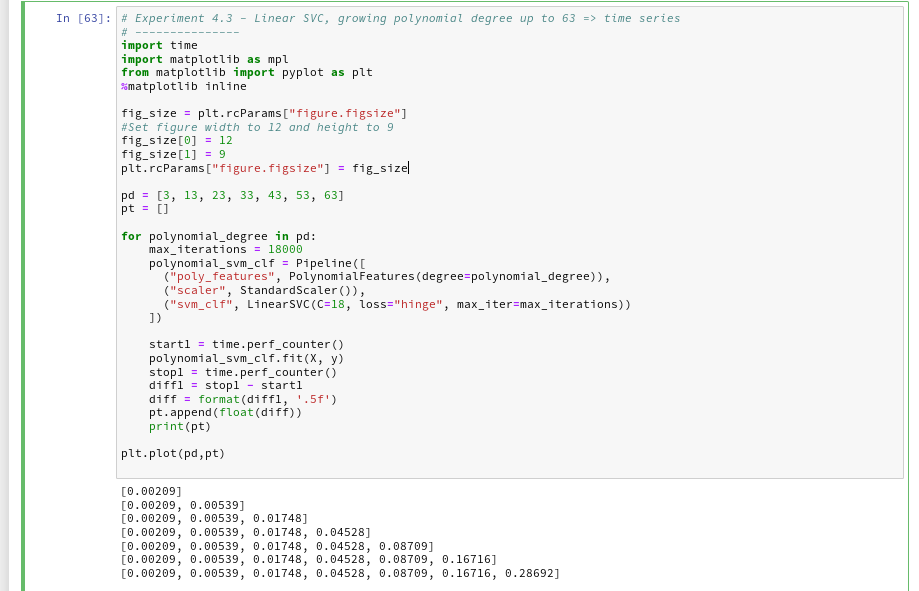And the plot looks like :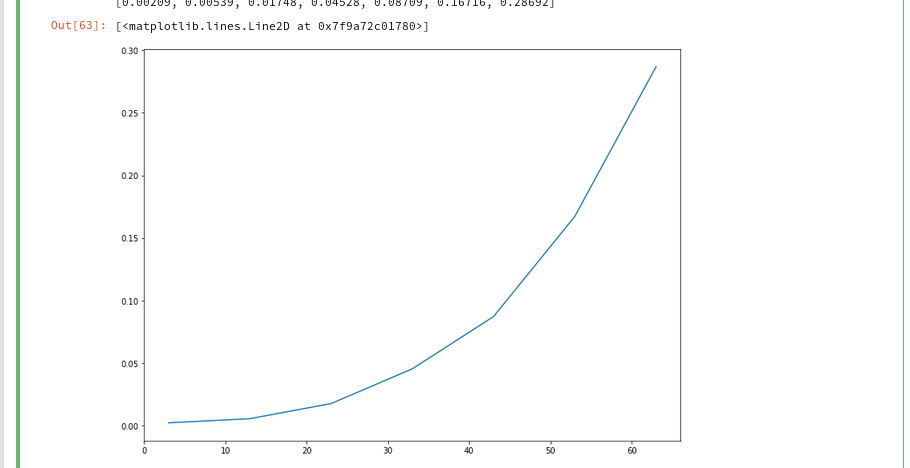We recognize the expected quadratic behavior.

# Polynomial kernel – almost constant low CPU-time independent of the polynomial degree

Let us now compare the output of the approach PolynomialsFeature + LinearSVC to an approach with the polynomial kernel. SciKit-learn provides us with an interface „SVC“ to the kernel based algorithms. We have to specify the type of kernel besides other parameters. We execute the code in the following 2 cells to get a comparison for a polynomial of degree 3: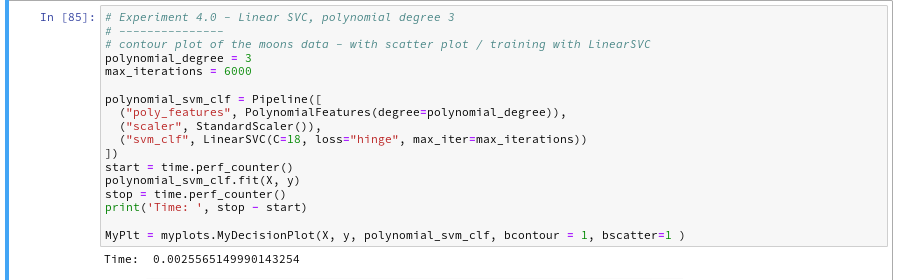You saw that we specified a kernel-type „poly“ in the second cell ?
The plots – first for LinearSVC and then for the polynomial kernel look like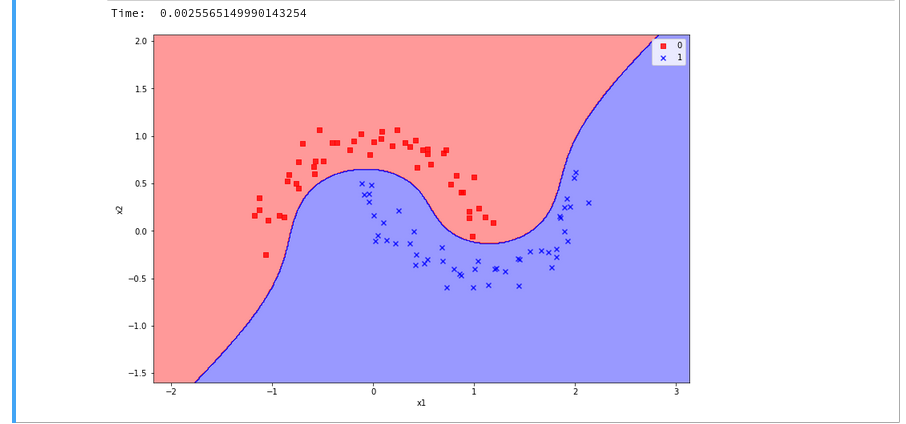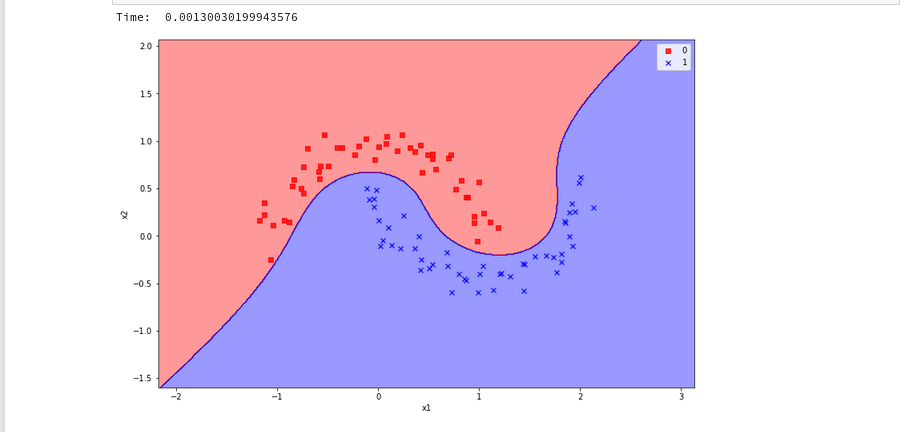We see a difference in the shape of separation line. And we notice already a slightly better performance for the kernel based algorithm.

Now let us prepare a similar time series for the kernel based approach: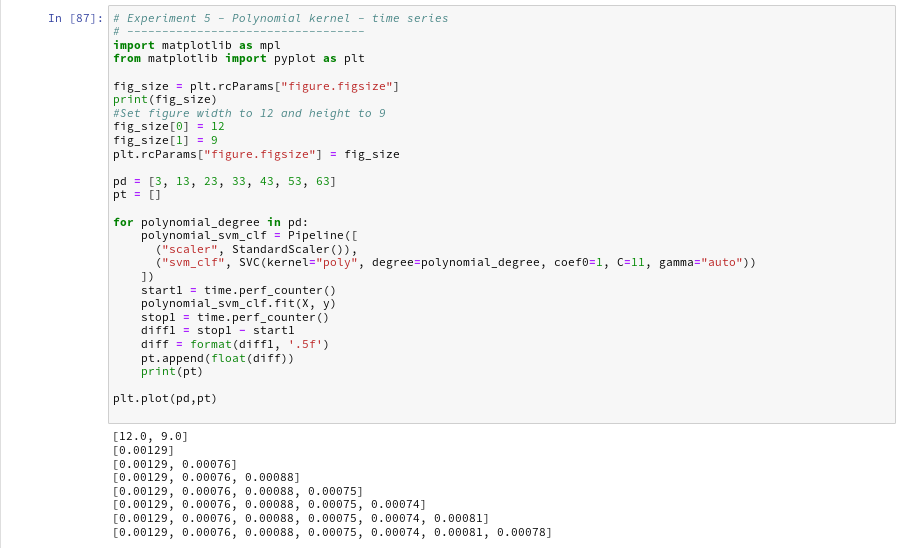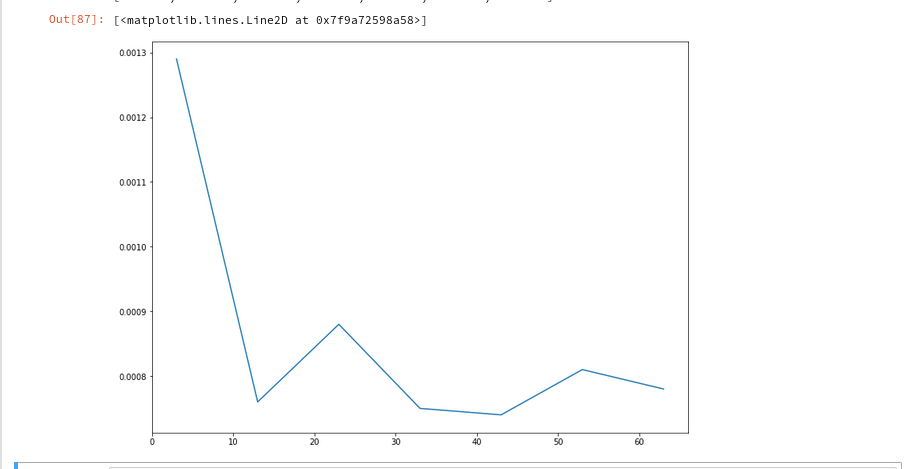The time series looks wiggled – but note that all numbers are below 1.3 msec ! What a huge difference!

# Plot for the Gaussian RBF Kernel

Just to check how the separation surface looks like for the Gaussian kernel, we do the following experiment; note that we specify a kernel named „rbf„: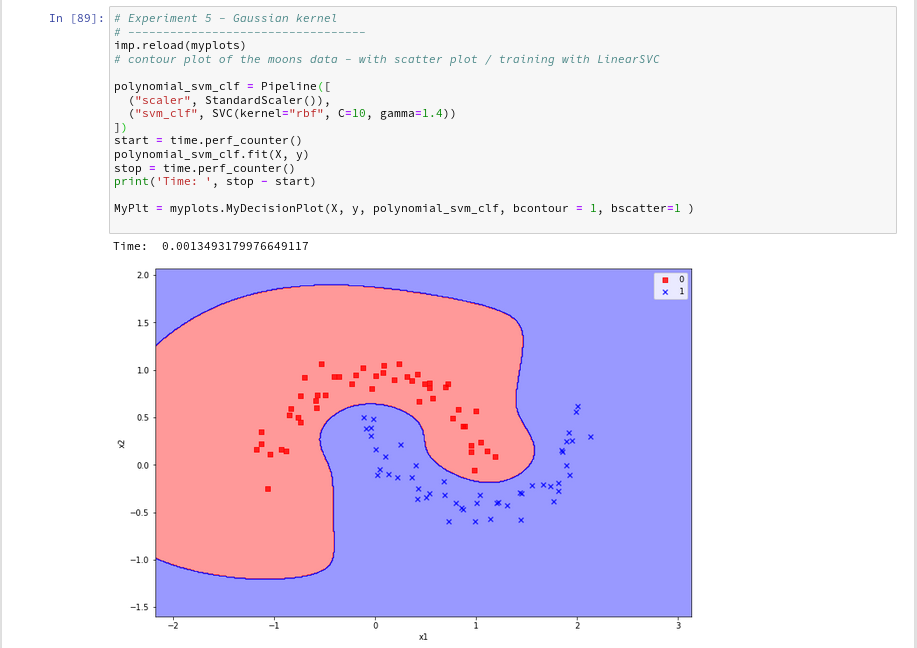Oops, a very different surface in comparison to what we have seen before.
But: Even a minimum change of gamma gives us yet another surface: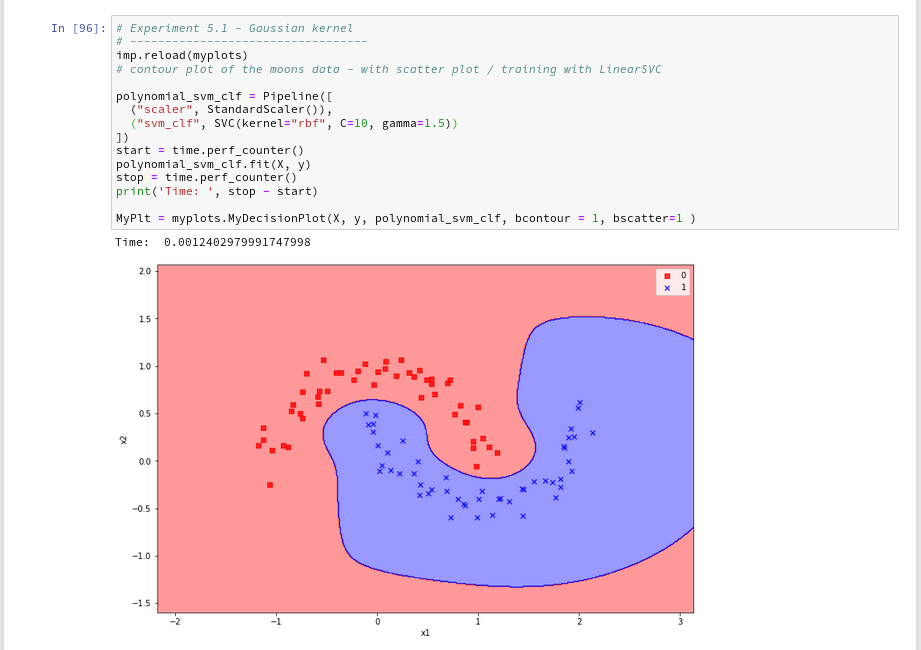Funny, isn’t it? We learn again that algorithms are sensitive!

Enough for today!

# Conclusion

We have learned a bit about the so called kernel trick in the SVM business. Again, SciKit-Learn makes it very simple for us to make use of kernel based algorithms via an SVC-interface: different kernels and related feature space extension methods can be defined as a parameter.

We saw that a “
poly“-kernel based approach in comparison to LinearSVC saves a lot of CPU-time when we use high order polynomials to extend the feature space to related higher dimensions.

The Gaussian RBF-kernel which extends the feature space by adding dimension based on weighted distances between data points proved to be interesting: It constructs a very different separation surface in comparison to polynomial approaches. We saw that RBF-kernel reacts sensitively to its configuration parameter „gamma“ – i.e the width of the Gaussian weighing the similarity influence of other points.

Again we saw that in regions of the (x1,x2)-plane where no test data were provided the algorithms may predict very different memberships of new data points to either of the two moon clusters. Such extrapolations may depend on (small) parameter changes for the algorithms.